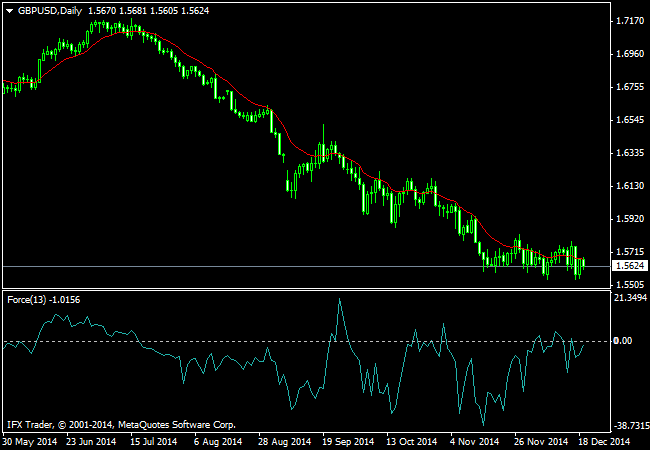# How to Use Force Index Indicator

Force Index Technical Indicator was developed by Alexander Elder. This index measures the Bulls Power at each increase, and the Bulls Power at each decrease. It connects the basic elements of market information: price trend, its drops, and volumes of transactions. This index can be used as it is, but it is better to approximate it with the help of Moving Average. Approximation with the help a short moving average (the author proposes to use 2 intervals) contributes to finding the best opportunity to open and close positions. If the approximations is made with long moving average (period 13), the index shows the trends and their changes.

In general, the force behind each price movement can be characterized by a direction, a movement size and a volume level. This force will be positive if the current bar’s close is above that of the previous bar. If it is lower, then the force has a negative sign.

Basically, the larger the movement from close to close, the higher the market’s force is. Also, the force also increases with higher trading volume.

Furthermore, since the Forex Index tends to swing considerably, traders often smooth out the data using a moving average that can be a simple, weighted or exponential moving average of the closing prices.

A common technique involves using 2 and 13-day exponential moving average to assess the strength of the short and medium-term trends respectively.

Sample Chart:#### How to Use Force Index Indicator in Trading

In practice, the Forex Index helps traders gauge the strength and direction of the prevailing trend. For example, some traders use the slope of the 13-day EMA Force Index to indicate the direction of the trend.

Furthermore, when the Forex Index is greater than zero, that indicates the trend is upwards, and when below zero, the trend is downwards. Nevertheless, when the Forex Index trades undecide around its zero point, this indicates a lack of trend in the market.

In terms of trading signals, traders might use the indicator to go long when the Force Index was under zero and bullish divergence appeared relative to the price. This means that the price makes new lows, but the Forex Index fails to do so.

Conversely, traders might go short when the Force Index was over zero and bearish divergence appeared relative to the price. This means that the price makes new highs, but the Index fails to do so.

Calculation Method:

#### Define:

• n = the number of the time period bar in question.
• N = the period of the smoothing using a moving average.
• Close(n) = The closing price at the end of time period n.
• Volume(n)= The volume traded during time period n.
• SMA(A,B) = Simple Moving Average of data item A over B periods.
• Forex Index (n)= The value of the Forex Index Indicator at time period n.

#### Calculate:

• Basic Force Index– Force Index(n) = [Close(n) – Close(n-1)] * Volume(n)
• Simple Smoothed Force Index– Force Index(n) = [SMA(Close(n), N) – SMA(Close(n-1), N)] * Volume(n)

#### How to Install Force Index Indicator On MT4 Charts

Force Index is a standard MT4 indicators, to install on your MT4 chart, please click on the menu  Insert  >>  Indicator >> Oscillator >> Force Index. In default setting Force Index is a period of 13 Exponenatial Moving Average and Apply to Close.

Tentang Penulis#### Syaifullah Chattar

CEO & Founder OmahForex, memulai trading forex pada pertengahan tahun 2005 .